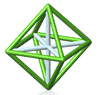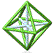Park City Mathematics Institute Secondary School Teacher Program Reflection on Practice Class: Teaching Through Problem Solving Akihiko Takahashi

Day One, Week Two - Monday, July 09, 2007

Aki talked about his workshop in Japan with teachers who visited Japanese schools. The teachers were asked what was interesting. Many mentioned the "Washlet" which was a combination toilet and bidet. In some way it was a solution to a problem that wasn't known in advance.

NCTM's View of Problem Solving:

1) problem solving means engaging in a task for which the solution method is not know in advance

These problems do not need to be story problems. They can be any problem where you don't know how to solve them.

The ones where kids know ahead of time how to solve them are called exercises

2) problem solving is an integral part of all mathematics learning, and so it should not be an isolated part of the mathematics program.

You can use problem solving to teach facts.

3) choosing worthwhile problems and mathematical tasks

There are many, many problems that are interesting and fun but may not lead to the development of the mathematical ideas that are important for a class at a particular time.

You need to be sure of what mathematics you want to teach. Order is critical. We need to have a specific goal of what the math is that we want to teach. It isn't true that a particular problem can be used across the curriculum.

Polya's 4 phases of problems solving work: A simplistic interpretation: Problem solving as an approach to develop problem-solving skills and strategies. Problem solving lessons are for developing problem solving skills and strategies. They often end when each student comes up with a solution to the problem (show and tell). They are isolated from the daily lesson. Here the lesson ends with the solution - but that shouldn't be the case. The important part is "after students find the answer - synthesizing all these ideas to get to higher math. " The goal is not, simply, to find answer. Real discussion starts after each student finds a solution.

PSSM "Teaching through problem solving"
Problem solving as a powerful approach for developing mathematical concepts and skills

Problem solving lessons - throughout the curriculum in order to develop mathematical concepts, skills, and procedures

Students' discussion becomes important

One of the focal points in the middle school is to write, interpret, and use mathematical expressions and equations to solve problems.

1) One of the challenges for the students is to write mathematical expressions that correspond to a given situation. Sometimes students may be reluctant to write math expressions because they often try to find the answer by simply carrying out calculations and cannot see the merits of writhing math expressions.

2) When designing such problem solving lesson, it is important to keep in mind that solving a problem is a process for providing an opportunity for students to appreciate that writing, interpreting and using a math expression. Therefore, the flow of lesson should not solely focus on finding the correct answer but also on the process of solving the problem.

An example of a worksheet for Problem Solving
You are selling ice cream form a cart. You sell ice cream bars for \$0.75 per box. Your cost for the ice cream is \$0.30 per bar and your cost for the rental of the cart is \$50.

a) In a formula, express your total cost C as a function of the number n of ice cream bars sold. On graph paper, graph C leaving room for negative values on the y-axis.

b) express the revenue R generated by the sale if ice cream bars as a function of the number n sold. Graph on the same graph as in a.

[There were two more parts.]

We are looking at this problem and asking whether this is really Problem Solving. These kids aren't constructing their own knowledge. They are given certain prompts. There was actually no question in these problems.

We see this sort of worksheet a lot. Why? Participant responses: We give them because we are worried that students won't be able to do it? We don't trust the mathematical knowledge they bring with them. We want them to get experience with these procedures. My objective for the day is normally not problem solving. Because, as teachers we aren't doing the research needed in order to write the books. This problem is designed to prepare the students for standardized testing. Here's a perfect time to scaffold - with multiple approaches. We are asking kids to generalize their arithmetical approach. If you ask a student to solve a problem 3 different ways, the student is going to ask why are you asking them to do it more than one way. We aren't trained to believe that is OK for kids to be frustrated. Remember that one of the definitions of "to cover" means "to obscure from view." Also, reading open ended problems is slow and difficult. This problem is more of a language exercise - using the letters C, r, t. This problem is like a car navigation system. By using this one lesson, we really expect our students to be able to solve similar problems. We give problems like this to be kind to our students.

We looked at the website: The Secret of the Crystal Ball. Ghost Whisperer

Choose any two digit number and subtract the sum of the digits from it: 57 - 12 = 45 Then see which symbol is associated with this number. Click on the Crystal Ball to see whether your symbol appears.

What sort of problems can you create using this site?

What mathematics can you teach? What kind of approaches will your kids take?

(10 a + b) - (a + b) = 9a

Why a multiple of nine? 82 - (8 + 2) = (82 - 2) - 8 = 80 - 8 = 10*8 - 8 = 9*8 so we'll always have a multiple of nine.

We'll always wind up with 9 times ten's digit.

We don't just want to end this lesson as show and tell.

What type of mathematics to we teach with this lesson? In order to do so, you need to anticipate student solutions.

100 a + 10 b + c = (a + b + c) = 99a + 9b is a multiple of 9, too.

Back to: Class NotesPCMI@MathForum Home || IAS/PCMI Home© 2001 - 2018 Park City Mathematics Institute IAS/Park City Mathematics Institute is an outreach program of the Institute for Advanced Study, 1 Einstein Drive, Princeton, NJ 08540 Send questions or comments to: Suzanne Alejandre and Jim King

With program support provided by Math for America

This material is based upon work supported by the National Science Foundation under DMS-0940733 and DMS-1441467. Any opinions, findings, and conclusions or recommendations expressed in this material are those of the author(s) and do not necessarily reflect the views of the National Science Foundation.